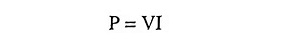## What is Voltage and Current?

There are two quantities that we like to keep track of in electronic circuits : voltage and current. These are usually changing with time; otherwise nothing interesting in happening.

Voltage (abbreviated as V or sometimes E) between two points is the cost in energy (work done) required to move a unit positive charge from the more negative point (lower potential to the more positive point (higher potential). Equivalently, it is the energy released when a unit positive charge moves “downhill” from the higher potential to the lower. Voltage is also referred to as potential difference (pd) or electromotive force (emf). Volt is its unit and voltages are usually expressed in volts, kilovolts (1 kV = 1,000 V), millivolts (1 mV = 0.001 V), or microvolts (1 μV = 10-6 V). A joule of work is required to move a coulomb of charge through a pd of one volt.

Current (abbreviated as I) is the time rate of flow of electric charge past a point. Ampere is its unit. The currents are usually expressed in amperes (A), milliamperes (1 mA = 0.001 A, microamperes (1 μA = 10-6 A), nanoamperes (1 nA = 10-9 A), or occasionally picoamperes (1 pA = 10-12 A). A current of one ampere is equal to the flow of one coulomb of charge per second. By convention, current in a circuit is considered to flow from higher potential to lower potential, even though the actual flow of electrons is in the opposite direction i.e. from lower potential to higher potential.

Voltages are produced by doing work on charges in devices such as batteries (electrochemical), generators electromagnetic forces), solar cells (photovoltaic conversion of the energy of photons), etc. Currents are produced by placing voltages across things like lamps, heating appliances, electric motors etc; called the load. In real circuits things are connected together with wires, metallic conductors.

The power consumed by an electric/electronic device is given asFor V in volts and I in amperes, power comes out in watts or joules per second (1 W = 1 J/s).

Power is usually expressed in watts, kilowatts (1 kW = 1,000 watts), milliwatts (1 mW = 10-3 W) or microwatts (1 μW = 10-6 W).

Power consumed is transformed usually into heat, or sometimes into useful mechanical work (motors), radiated energy (lamps, radiators or transmitters), or stored energy (capacitors, batteries).

Scroll to Top